Back to Nautical Definition Charts Rotation of the Earth Distances Position Lines Sailings Chart Work Exercises Information from Charts Tides Sextant

Sailings

OBTAINING VESSELS POSITION USING CELESTIAL OBSSERVATIONS

Position finding at sea by the methods of nautical astronomy depends upon the solution of an oblique spherical triangle, and practice in the application of the formula is essential in order to acquire facility in its manipulation.

In the following figure, the triangle ABC is a spherical triangle. It has been formed by the intersection of three great circles. Great circles are those whose planes pass through the centre of the sphere.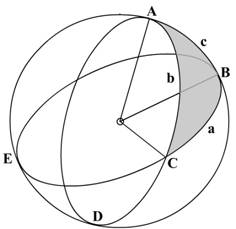ABD, ACP and BCE are three great circles, and triangles ABC and DCB are two of the spherical triangles formed by their intersection. All the angles at A, B and C are spherical angles.

As has been stated before the sides of a spherical triangle are measured and expressed in angular notation instead of in distance units. However on the surface of the Earth, the sides are converted from the angular measurement into the distance unit by multiplying with the length of the ONE-MINUTE of the arc, that is 1853 nautical miles.

The chief properties of a spherical triangle are:

The sides are expressed in angular measure

The sum of all the angles in a spherical triangle is equal to a figure between 180° to 540° or between 2 right angles to six right angles.

The sum of the three sides of a spherical triangle must be less than 860°

As with plane triangles the greatest side is opposite to the greatest angle.

Where 2 great circles cut each other the vertically opposite angles are equal.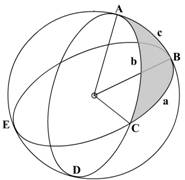In the above figure, we have the angles as A, B and C, the sides corresponding to the angles would be a, b and c

The formula used to calculate an angle or a side is given by:

hav A   = hav a – hav (b~c)

sin b x sin c

In the above formula the expression hav (b~c) indicates that the result should always be positive. In case b is greater than c, then c will be subtracted from b, and vice versa. The symbol ‘~’ indicates difference.

In the above we have tried to find the angle A, however if the angle required is B then the formula has to be replaced by the known figures.

hav B   = hav b – hav (a~c)

sin a x sin c

The formula would again have to be amended if any of the sides are required.

Solution Of Right Angled Spherical Triangles

A rare triangle since it is not always possible to get a right angled spherical triangle.

The right-angled spherical triangle may be solved using the haversine formula as described above but may also be solved easily using Napier’s Rules for Circular Parts.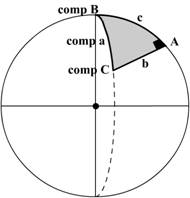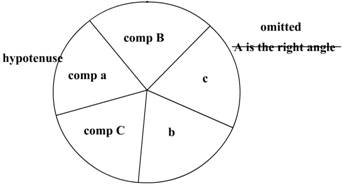In the above figure the right angled spherical triangle is shown, the hypotenuse and the other sides are indicated.

The rules are thus:

A triangle has six parts – the three sides and the three angles. However using Napier’s Rule the 5 parts are used, the right angle is omitted.

The complements of the hypotenuse and the two angles are used. Complement means that the angles have to be subtracted from 90° and then used. However instead of subtracting from 90° we may also use the following:

sine (90°- Angle) = cosine angle

tangent (90° - angle) = cotangent angle

Adjacent part means the immediate next part. Opposite means the part next to an adjacent part.

If any of the three parts is selected as a mid part, the other two must be either adjacent parts or opposite parts. This depends on which formula is used as stated below:

Sine of middle part = product of tangents of adjacent parts

OR

Sine of middle part = product of cosines of opposite parts.

Filling up the circular part, start from any angle or side and proceed in a clockwise direction similar to the triangle itself.

REMEMBER THAT THE COMPLEMENTS ARE USED OF THE HYPOTENUSE AND THE TWO ANGLES – THE RIGHT ANGLE IS OMITTED.

THERE ARE FIVE PARTS AND NOT SIX.

Sailings

The compass was developed about 1000 years ago. However it was not until the 1870s that Lord Kelvin developed a reliable dry card marine compass. The fluid-filled compass became standard in 1906.

Variation was not understood until the 18th century, when Edmond Halley led an expedition to map lines of variation in the South Atlantic. Deviation was understood at least as early as the early 1600s, but correction of compass error was not possible until Matthew Flinders discovered that a vertical iron bar could reduce errors. After 1840, British Astronomer Royal Sir George Airy and later Lord Kelvin developed combinations of iron masses and small magnets to eliminate most magnetic compass error.

The gyrocompass was made necessary by iron and steel ships. Leon Foucault developed the basic gyroscope in 1852. An American (Elmer Sperry) and a German (Anshutz Kampfe) both developed electrical gyrocompasses in the early years of the 20th century.

Coordinates, termed latitude and longitude, can define any position on earth.

Latitude (Lat.) is the angular distance from the equator, measured northward or southward along a meridian from 0°at the equator to 90°at the poles.

It is designated north (N) or south (S) to indicate the direction of measurement.

The difference of latitude (DLat.) between two places is the angular length of arc of any meridian between their parallels.

It is the numerical difference of the latitudes if the places are on the same side of the equator; and

It is the sum of the latitudes if the places are on opposite sides of the equator.

It may be designated north (N) or south (S) when appropriate.

The middle or mid-latitude (midLat.) between two places on the same side of the equator is half the sum of their latitudes. Mid-latitude is labeled N or S to indicate whether it is north or south of the equator.

The expression may refer to the mid-latitude of two places on opposite sides of the equator.

When the places are on opposite sides of the equator, two mid-latitudes are generally used.

Calculate these two mid-latitudes by averaging each latitude and 0°

Longitude (Long.) is the angular distance between the prime meridian and the meridian of a point on the earth, measured eastward or westward from the prime meridian through 180°.

It is designated east (E) or west (W) to indicate the direction of measurement.

The difference of longitude (DLong) between two places is the shorter arc of the parallel or the smaller angle at the pole between the meridians of the two places.

If both places are on the same side (east or west) of Greenwich, DLong is the numerical difference of the longitudes of the two places; if on opposite sides, DLong is the numerical sum unless this exceeds 180°, when it is 360°minus the sum.

The distance between two meridians at any parallel of latitude, expressed in distance units, usually nautical miles, is called departure

(Dep.). It represents distance made good east or west as a vessel proceeds from one point to another.

Its numerical value between any two meridians decreases with increased latitude, while Dlong. is numerically the same at any latitude.

Either DLong. may be designated east (E) or west (W) when appropriate.

Distance

Distance, as used by the navigator, is the length of the rhumb line connecting two places. This is a line making the same angle with all meridians. Meridians and parallels, which also maintain constant true directions, may be considered special cases of the rhumb line. Any other rhumb line spirals toward the pole, forming a loxodromic curve or loxodrome.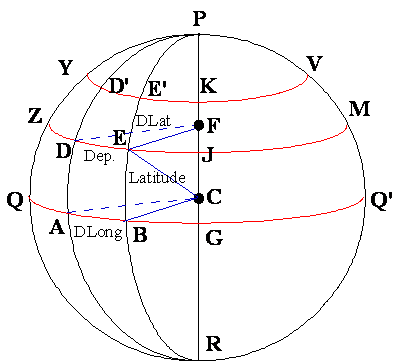AB and DE are arcs of concentric circles, if viewed from the poles, their centres F and C being on the earth’s axis, and as arcs of circles are proportional to their radii, thus we have: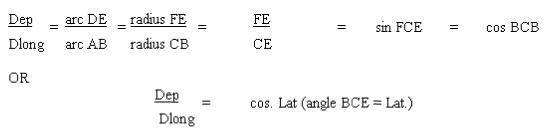The value that is required may be now found using the above formula and transposing as required.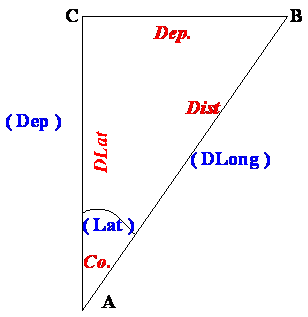In the above simple right angled plane triangle, the Blue Regular font indicates the factors used in the same triangle in the Travers Tables for using in Parallel sailing problems.

The Red Italics font indicates the factors used in the Traverse Tables for solving problems in Plane sailing.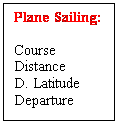In PLANE SAILING the angle is made equal to the Course.

The hypotenuse is made equal to the Distance.

The side adjacent to the angle is made equal to the D. Latitude.

And the side opposite to the angle is made equal to the Departure.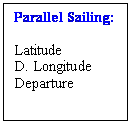In PARALLEL SAILING the angle is made equal to the Latitude.

The hypotenuse is made equal to the D. Long.

And the side adjacent to the angle is made equal to the Departure.

The Traverse tables are a combination of the two tables as above, the navigator must be careful to select the correct table. The entries are as described above. The Italics and Regular font play an important part in differentiating the Tables.

Finding the Difference in Latitude (D.Lat.) and the Difference in Longitude (D.Long).

(Before proceeding with examples in parallel sailing problems)

D. Lat.: Subtract the two latitudes if they have the same names.

Add if the two Latitudes have different names.

The naming convention is Northerly D.Lat if more North and Southerly if more South.

Once the D.Lat in degrees and minutes has been found out – convert the same into minutes. D. Lat is then used as minutes.

D. Long: Subtract the two Longitudes if they have the same names.

Add if the two Longitudes have different names.

The naming convention is Easterly D. Long if more East and Westerly if more West. If the sum of a East longitude or a West longitude exceed 360˚, then the same is subtracted from 360˚ and the name is reversed.

Once the D. Long in degrees and minutes has been found out – convert the same into minutes. D. Long is then used as minutes.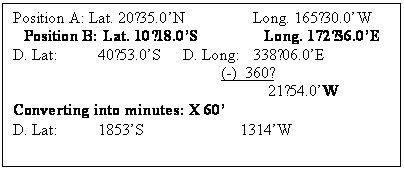PARALLEL SAILING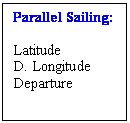As explained before the factors are: Latitude, D. Longitude and the Departure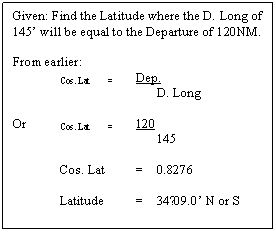Parallel sailing as stated above has lost its prominence in navigation. This type of sailing is no longer practiced, however it is still used for finding out the mean distance traveled between two meridians.

Used for distances less than 600 NM, the parallel sailing formula is used with a difference.

The Latitude used is that of Mean between the two positions.

So now the formula stands amended to:

Dep. = D. Long x cos. Mean Latitude.

As long as the difference between the Latitudes is small, Mean Latitude used is sufficient, however if the Difference of Latitude is large then, Middle Latitude is used.

Mean Latitude is the arithmetical mean of the two latitudes, but Middle Latitude takes into account the convergence of the earth.

Middle Latitude is the Latitude on which the actual Departure lies.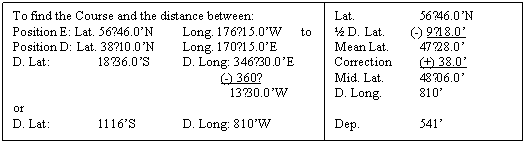This convergence correction is obtained from the nautical Tables.

Knowing the departure we can now find the Course and the Distance.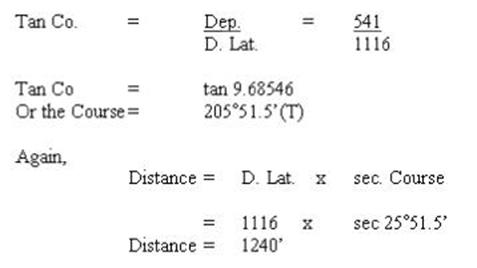Mercator Sailing gives accurate results for long distances, more so when there is a very large change in the Latitude between the two positions.

Finally:

Mercator Sailing:          Course D. Long.           DMP (difference in Meridional Parts)

Parallel Sailing:  Mid. Lat.          D. Long.           Dep.

Plane Sailing:    Course Distance           Dep.     D. Lat.

The connections:

D. Long. Connects Mercator to Parallel Sailing

Departure connects Plane with Parallel Sailing

Course connects Mercator with Plane Sailing.

Using the above we can find out the set and drift of the current, or knowing the set and drift of the current we can find our DR position. This is useful if we have to make a rendezvous with another vessel in a search and rescue operation.

 DR Posn.:    Lat.:  50°20.0’N Long.: 115°37.0’E Obs. Posn:   Lat.:  50°40.0’N Long.: 115°20.0’E To find the set and drift. DR Posn.:    Lat.:  50°20.0’N Long.: 115°37.0’E Obs. Posn:   Lat.:  50°40.0’N Long.: 115°20.0’E D. Lat:                    00°20.0’N D. Long: 00°17.0’ Use Traverse Tables: M. Lat.: 50°30.0’ & D. Long Gives a Dep.: 10.8 Entering with D. Lat & Dep. Gives: Course:      28°              Distance: 23’ So the set would be N28°W and the Drift would be: 23NM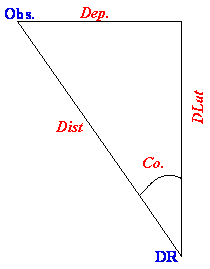The Meridional Parts for any latitude is the increased length of the meridians on a Mercator chart measured from the equator to the parallel and expressed in minutes of the Longitude scale.

Example: The length of the meridian A to D when measured along the equator represents the number of meridional parts corresponding to ad; and AE to ae; AF to af and so on.

If position d is Latitude 30°N, then the measured as a line distance on the earth’s surface of ad is 30° x 60’ = 1800NM, but on referring to the Meridional Parts table in the Nautical Tables the MP for 30° is 1877, so the meridional distance AD on the chart is 77 equatorial minutes longer than on the globe.

Difference Of Meridional Parts:

Is the difference between the MP of two Latitudes.

Using MP the factor Departure disappears and is replaced by D. Long.

In the same equation Latitude also disappears and is replaced by DMP.

So on the Mercator chart we would have: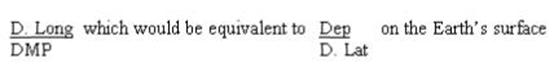The Navigational features of a Mercator Chart are:

The world, with the exception of the higher Polar Regions, can be seen at a glance

The rhumb line track from one position to another is a straight line

The great circle track from one position to another is an arc.

The course from one position to another can be read by using a parallel ruler and playing it to the compass rose and reading the course directly.

The scale of the Latitude at the side of the chart is also the scale for distance in nautical miles. Note however that if the positions are separated by a considerable difference in Latitude then the divider for measuring the distance should be placed at the mean latitude or they will be a discrepancy.

Calculating the course and distance between two places is reduced to solving a plane right-angled triangle.

The Great Circle track is the shortest distance between two places. (When a crow flies or when a person is traveling between two points that are visible on the earth’s surface but separated by some distance, the person makes a GC track.)

However if sailing on a Mercator chart and following a GC track the course line would be a curve as such the vessel would have to be continually altering course, this because the track is crossing the meridians at a different angle. (An aircraft pilot on the other hand when he does visual navigation instead of following a charted course line is doing a GC track).

The GC track bends towards the Pole, so on a East-West course the course line would initially be NE then E after passing the vertex the course would become slowly SE.

Since the course cannot be continually altered, an alternate method is chosen. The course line is laid on a Gnomonic Chart, where the GC track would appear as straight line.

From this the alteration points are decided, say at every 10° of longitude, the co-ordinates (Latitude and Longitude) of these points are taken and are transferred to a Mercator Chart.

And the ship then sails a rhumb line track between the selected parts of th4e track, altering as and when she reaches the alteration points.

Mercator Sailing:

The above calculations are resorted to when a distance of more than 600NM separates the two positions.

Example:

 To find the Course and Distance between: Posn A: Lat.: 49°58.0’N       MP: 3453.43       Long.: 005°12.0’W Posn B: Lat.: 37°49.0’N       MP: 2439.97       Long.: 025°08.0’W     For the calculation we add a column for MP A: Lat.: 49°58.0’N                 MP: 3453.43       Long.: 005°12.0’W B: Lat.: 37°49.0’N                 MP: 2439.97       Long.: 025°08.0’W D. Lat.: 12°09.0’                 DMP: 1013.46       D. Long.: 19°56.0’ D. Lat.: 729’                                                       D. Long.: 1196’   In the triangle ABC we have: B = 90°, AB = DMP, BC = D. Long We have to find A – the course steered. Tan course = D. Long              =        1196                          DMP                   1013.5 Course   = 49°43.3’                     or S49°43.3’W      or   229°43.3’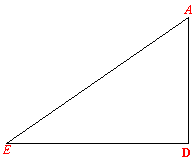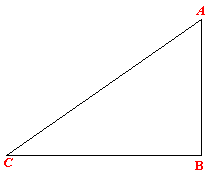Once the Course is obtained, we use the course to find the distance sailed.   AD          =              D. Lat     =              729 A             =              49°43.3’ D             =              90° In the triangle ADE: AE          =              AD x sec. A           or Distance                =              D. Lat. x secant A Or            =              729 x sec. 49°43.3’ Distance                =              1128NM

NOTE: THE LINE AC IS NOT THE DISTANCE FROM POSITION. A, TO POSITION B, IT IS SIMPLY A RHUMB LINE TO COMPLETE THE TRIANGLE.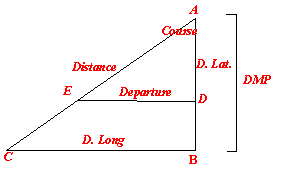From the above example, if we plot the factors, we would obtain 2 right-angled triangles. They both have in common are the Course and the distance steamed.

The above shows how the two equations may be merged to get the course and the distance between two points.

GREAT CIRCLE SAILING

Due to the Earth being almost a sphere the shortest distance as measured on the Earth’s surface would be a straight line that would curve with the curvature of the Earth.

Since all navigation is however done on a Mercator chart which has been designed to show the surface of the Earth as a flat piece of paper – the straight line that is drawn on the chart is not a straight line on the surface of the Earth.

There lies therefore a difference in the planning of the sailing on paper and the final sailing itself which is done on the surface of the Earth.

For short distances and sometimes for convenience the plane and parallel sailing is utilized, however when undertaking long trans Pacific or Atlantic sailings a great circle route reduces the distance by an appreciable amount.

Due to the nature of the Mercator chart a great circle course (straight line on the surface of the Earth) is produced as a curve. Again a difficulty arises as to how to draw the curve.

Therin comes the requirement of the calculation of the Great circle track – the initial course – the final course and the overall distance. Once these are found out the nest step is to follow this curved course line on a Mercator chart. Obviously a ship cannot keep altering her course to follow the curve so a interval of say 10° is adopted and the curve is broken up into a series of short straight lines on the Mercator chart, these short straight lines would follow the curve as much as possible.

If however a gnomonic chart (the chart is as if it has been peeled off from the surface of the Earth – the poles are as they should be) is available on board then the great circle track may be drawn as a straight line from the initial position to the final position. This line as already explained would be a curve on the Mercator chart.

As discussed earlier the interval of 10° is adopted and the points of alteration of course is lifted from the gnomonic chart and the positions are transferred to the Mercator chart and the straight lines are joint to the points so obtained (at intervals of 10°). Thus a modified curve is finally sailed on.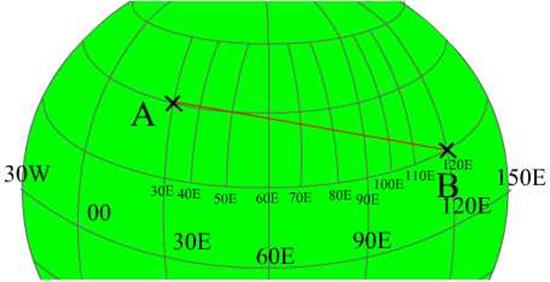All calculation of the initial course, final course as well as the distance between poits ‘A’ and ‘B’ are resolved using the haversine formulae for spherical triangles. Since on the surface of the Earth the sides of the triangle are made up of great circles.

Thus, the formula used is:

Haversine (one side) = haversine (opposite angle) x sine (adjacent side) x sine (adjacent side) + haversine (difference between adjacent sides).

Coming to and example: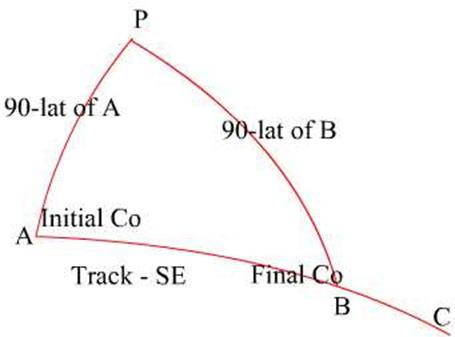In the above triangle the distance as required is from point A to point B.

So,

Hav AB = hav P x sin PA x sin PB + hav (PA-PB)

Where PA is the meridian passing through point ‘A’ and PB is the meridian passing through ‘B’ and AB is the intended track.

Let us assume that point A and B have the following co-ordinates:

 Latitude Longitude Initial: A 20°00’N 030°00’E Final: B 10°00’N 120°00’E Difference 10°00’N (d.lat) 90°00’E (d.long)

Since the travel from ‘A’ to ‘B’ is eastwards the difference is East, the naming is irrespective of the longitude – it depends only on the track direction.

Therefore: Hav AB = hav P x sin PA x sin PB + hav (PA-PB)

PA is co-lat of A = (90-20) = 70°, and PB is the co-lat of B = (90-10) = 80°

Inserting the values to the equation:

Therefore: Hav AB = hav P x sin PA x sin PB + hav (PA-PB)

Hav AB = hav (d.long) x sin (90-lat of A) x sin (90-lat of B) + hav (d.lat)

Hav AB = hav 90° x sin 70° x sin 80° + hav 10°

AB = 86°35.7’ equates to a distance of 5195.7 NM

Calculating the Initial course for the above example:

We now have the triangle PAB with all the three side values and the value of the angle P.

It thus becomes easy to apply the haversine formula to get the other values.

To find the initial course we have to find the value of angle at A (angle PAB), using the formula:

Haversine of one angle = cosecant adjacent side x cosecant adjacent side x (haversine opposite side – haversine difference between the adjacent sides)

Or applying to our figure: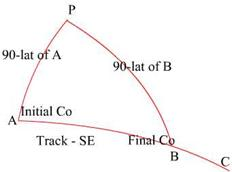Hav A = cosec PA (cosecant adjacent side to A) x cosec AB (cosecant adjacent side to A) x [hav PB (haversine opposite side to A) – hav (PA~AB) {haversine difference between the adjacent sides}]

Hav A = cosec PA x cosec AB x [hav PB – hav (PA~AB)]

Inserting the values:

Hav A = cosec 70 x cosec 86°35.7’ x [hav 80 – hav (86°35.7’-70)]

Hav A = cosec 70° x cosec 86°35.7’ x hav 63.4°

A = 80°35.5’SE equates to 099°24.5’ = Initial Course

Calculation of the Final Course:The Final Course is the angle PBC, so in order to get that angle we would have to find the angle PBA and then subtract or add 180° to it.

Thus by haversine formula we need to obtain the angle PBA.

Again:

Hav B = cosec PB (cosecant adjacent side to A) x cosec AB (cosecant adjacent side to A) x [hav PA (haversine opposite side to B) – hav (PB~AB) {haversine difference between the adjacent sides}]

Hav B = cosec PB x cosec AB x [hav PA – hav (PB~AB)]

Inserting the values:

Hav B = cosec 80 x cosec 86°35.7’ x [hav 70 – hav (86°35.7’- 80)]

Hav B = 70°16.8’ equates to 109°43.2’ = Final Course

Composite great circles

We have previously calculated distances using the great circle method, no doubt there would be a great saving in distance. But what happens if the Great Circle track takes a ship to extreme high latitudes where the weather associated with the latitude is – too cold and too rough?

To escape such weather the idea is to sail part of the way on a Great Circle track and then sail along a parallel and then again do a Great Circle sailing until the destination point is arrived at. This type of sailing is known as Composite Sailing.

So how is this done?

The initial position is taken and the vessel sails up to the latitude of the Vertex and then sails along the parallel of latitude of the Vertex and then after sailing thus sails down to the final point in a Great Circle track.

This brings up Vertex.

Vertex of a great circle as stated above is the point where the Great Circle reaches its maximum latitude that the vessel has decided on.

A Great Circle would have 2 Vertex’s, 180° apart. That is one in either hemisphere.

A Great Circle track extended to cut the equator at any point would have a difference of longitude of 90° between that point and the Vertex.

For a Great Circle as the Great Circle goes away from the equator the North or the South component of the track progressively reduces and the East or West component keeps increasing. This is so until the Vertex is reached where the North or South component completely disappears and the East or the West component is the only one present.

After the vertex the track slowly gains the North or South component and loses the East or West component until the equator.

The Vertex gives warning of the extreme latitude that the vessel may reach while sailing the Great Circle track. So during planning the track may be modified to complete the sailing on favourable maximum latitude. The position of the Vertex also eases the calculation of the intermediate position of the track (as decided for using the track on a Mercator Chart).

Position of the Vertex:

01.  Vertex is in the same hemisphere if both the Initial and the Final positions are in the same hemisphere.

02.  Vertex is in the hemisphere of the higher latitude (between the Initial and Final points) if they lie in separate hemispheres.

03.  The Vertex lies between the Initial and the Final points if both the points are in different quadrants (NESW).

04.  However if the Initial and the Final points are both in the same quadrant then the Vertex would lie on the extended track joining the two, either towards the Initial point or towards the Final point.

In the example used until now the Vertex would be as such: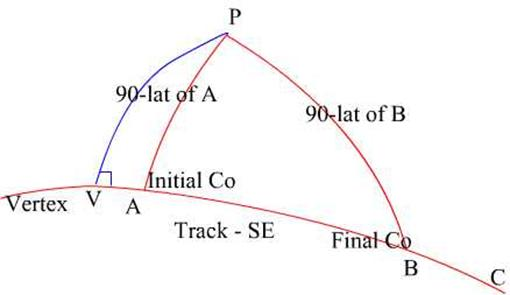Consider the triangle PAV, where the point V is the Vertex and a right angled spherical triangle, since PV is the meridian of V. The course line would be East or West in the example it is Easterly.

Then using Napiers rule: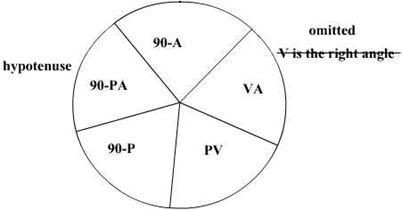To find the latitude of the Vertex:

From Napiers Rule:

Sine of mid part = cosine of opposite parts or

Sin mid part = cos opp part x cos opp part

Sin PV = cos (90 – A) x cos (90 – PA)

Sin PV = cos (90 - 80°35.5’) x cos (90 – 70)

PV = 67°59’ or the Latitude of the Vertex is 22°01’N

To find the Longitude:

Sin (90 - PA) = tan (90 - A) x tan (90 - P) or

Tan (90 – P) = sin (90 – PA) x 1/ tan (90-A)

Tan (90 – P) = sin (90 – 70) x 1/ tan (90 - 80°35.5’)

Tan (90 – P) = tan P = 64°09.2’West of A, therefore the Longitude of the Vertex would be: 034°09.2’W

Now to move on to Composite Great Circle sailing,

Point to remember is that there are actually 2 Great Circles, one for the initial course and upto the Vertex latitude and then there is parallel sailing along the latitude of the Vertex and then there is the other Great Circle which would bring the vessel to the destination – the final course.

Basically since the Great circles have been separated and one part of the triangle is a right angled triangle (due to the Vertex) the entire exercise is done using Napiers Rule and so is less time consuming.

The figure shows the two Vertex’s of the two Great Circles, and the parallel track between the two Vertex’s.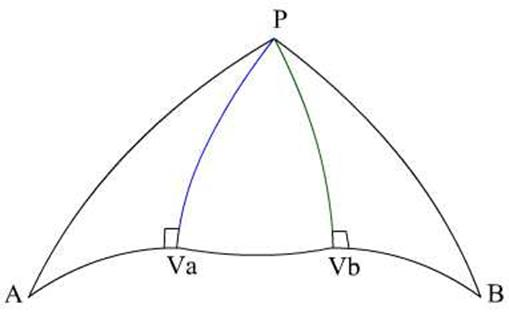Given a vessel would like to have the maximum latitude during a Great Circle passage to be 55°N. Given that the vessel would depart from position:

 Latitude Longitude Initial – A 49°°00’N 030°00’E Final - B 50°00’N 120°00’E Difference 90°00’E (d.long)

Using Napiers RuleLat A 49N PB 40° Lat B 50° Lat Va 55N PW 35° PB 40 PA 41° PV 35°

In the first right angled spherical triangle PAVa

Sin A = cos lat Va x Sec lat A

Sin A = cos 55° x sec 49°

A = 60°57.6’ Initial Course

Again for the distance AVa:

Cos AVa = sin lat A / sin lat Va

Cos AVa = sin 49 / sin lat 55

AVa = 1372.7 NM

In the final right angled triangle PBVb:

Sin B = cos lat Vb x Sec lat B

Sin A = cos 55° x sec 50°

A = 116°10’’ Initial Course

Again for the distance AVb:

Cos AVb = sin lat B / sin lat Vb

Cos AVb = sin 40 / sin lat 55

AVb = 2298 NM

Once the Great Circle tracks have been covered, the parallel sailing track has to be calculated – that is the distance between Va to Vb.

For this the longitude of the two Vertex’s have to be found first.

For triangle PAVa:

Cos P = Tan lat A / tan lat Va

Cos P = tan 49 / tan 55

P or the d.long between A to Va = 36°20.5’E

Therefore the Longitude of the First Vertex (Va) would be:

Longitude of A + d.long = 30°E + 36°20.5’ = 66°20.5’

For triangle PAVb:

Cos P = Tan lat B / tan lat Vb

Cos P = tan 50 / tan 55

P or the d.long between B to Vb = 33°26.3’

Therefore the Longitude of the First Vertex (Vb) would be:

Longitude of B - d.long = 120°E - 33°26.3’ = 86°33.7’

D.long between the longitudes of Va and Vb

D long: 86°33.7’ - 66°20.5’

Distance VaVb = dep = d.long x cos lat

Distance = 688NM

So adding up all the distances: 1327 + 2298 + 688 = 4313 NM